# ML Aggarwal Class 7 Solutions for ICSE Maths Chapter 11 Triangles and its Properties Objective Type Questions

## ML Aggarwal Class 7 Solutions for ICSE Maths Chapter 11 Triangles and its Properties Objective Type Questions

Mental Maths

Question 1.
Fill in the blanks:
(i) A triangle has at least ………… acute angles.
(ii) A triangle cannot have more than ……… right angle.
(iii) A triangle cannot have more than ………… obtuse angle.
(iv) In every triangle, the sum of (interior) angles of a triangle = ……….. right angles.
(v) In every triangle, an exterior angle + adjacent interior angle = ………… degrees.
(vi) In every triangle, an exterior angle = sum of the …….. interior opposite angles.
(vii) In a right-angled triangle, if one of the acute angles measures 25° then the measure of the other acute angle is …………
Solution:Question 2.
State whether the following statements are true (T) or false (F):
(i) A triangle can have all three angles with a measure greater than 60°.
(ii) A triangle can have all three angles with a measure of less than 60°.
(iii) If an exterior angle of a triangle is a right angle, then each of its interior opposite angles is acute.
(iv) In a right-angled triangle, the sum of two acute angles is 90°.
(v) If all the three sides of a triangle are equal, then it is called a scalene triangle.
(vi) Every equilateral triangle is an isosceles triangle.
(vii) Every isosceles triangle must be an equilateral triangle.
(viii) Each acute angle of an isosceles right-angled triangle measures 60°.
(ix) A median of a triangle always lies inside the triangle.
(x) An altitude of a triangle always lies outside the triangle.
(xii) In a triangle, the sum of squares of two sides is equal to the square of the third side.
Solution: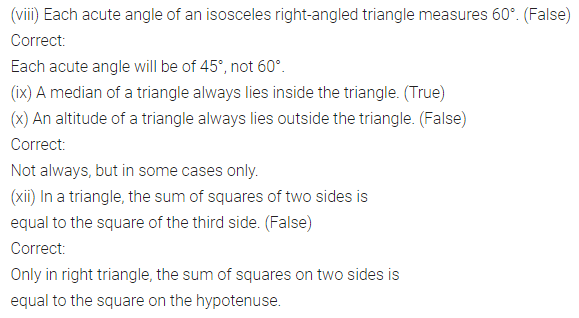Multiple Choice Questions

Choose the correct answer from the given four options (3 to 17):
Question 3.
A triangle formed by the sides of lengths 4.5 cm, 6 cm, and 4.5 cm is
(a) scalene
(b) isosceles
(c) equilateral
(d) none of these
Solution:Question 4.
The number of medians in a triangle is
(a) 1
(b) 2
(c) 3
(d) 4
Solution: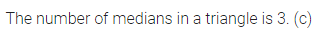Question 5.
An exterior angle of a triangle is 125°. If one of the two interior opposite angles is 55° then the other interior opposite angle is
(a) 70°
(b) 55°
(c) 60°
(d) 80°
Solution:Question 6.
In a ∆ABC, if ∠A = 40° and ∠B = 55° then ∠C is
(a) 75°
(b) 80°
(c) 95°
(d) 85°
Solution: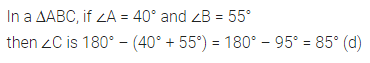Question 7.
If the angles of a triangle are 35°, 35°, and 110°, then it is
(a) an isosceles triangle
(b) an equilateral triangle
(c) a scalene triangle
(d) right-angled triangle
Solution:Question 8.
A triangle whose two angles measure 30° and 120° is
(a) scale
(b) isosceles
(c) equilateral
(d) none of these
Solution: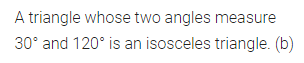Question 9.
A triangle can have two
(a) right angles
(b) obtuse angles
(c) acute angles
(d) straight angles
Solution: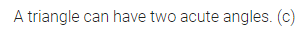Question 10.
A triangle whose angles measure 35°, 35° and 90° is
(a) acute-angled
(b) right-angled
(c) obtuse-angled
(d) isosceles
Solution: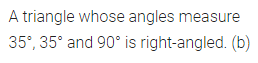Question 11.
A triangle is not possible whose angles measure
(a) 40°, 65°, 75°
(b) 50°, 56°, 74°
(c) 72°, 63°, 45°
(d) 67°, 42°, 81°
Solution:Question 12.
If in an isosceles triangle, each of the base angles is 40°, then the triangle is
(a) right-angled triangle
(b) acute-angled triangle
(c) obtuse-angled triangle
(d) isosceles right-angled triangle
Solution:Question 13.
A triangle is not possible with sides of lengths (in cm)
(a) 6, 4, 10
(b) 5, 3, 7
(c) 7, 8, 9
(d) 3.6, 5.4, 8
Solution: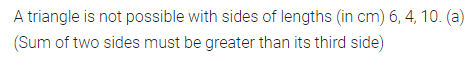Question 14.
Which of the following can be the length of the third side of a triangle whose two sides measure 18 cm and 14 cm?
(a) 32 cm
(b) 3 cm
(c) 4 cm
(d) 5 cm
Solution:Question 15.
In a right-angled triangle, the lengths of two leg’s are 6 cm and 8 cm. The length of the hypotenuse is
(a) 14 cm
(b) 10 cm
(c) 11 cm
(d) 12 cm
Solution: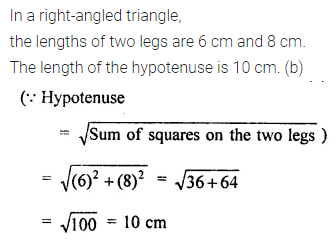Question 16.
If the dimensions of a rectangle are 15 m and 8 m, then the length of a diagonal is
(a) 7 m
(b) 23 m
(c) 17 m
(d) 20 m
Solution:Question 17.
If p, q, and r are the lengths of the three sides of a triangle, then which of the following statements is correct?
(a) p + q = r
(b) p + q < r (c) p + q > r
(d) p – q > r
Solution: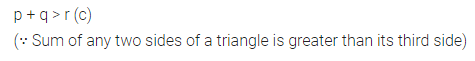Higher Order Thinking Skills (HOTS)

Question 1.
In ∆DEF, DM and EN are two medians. Prove that 3(DF + EF) > 2(DM + EN).
Solution: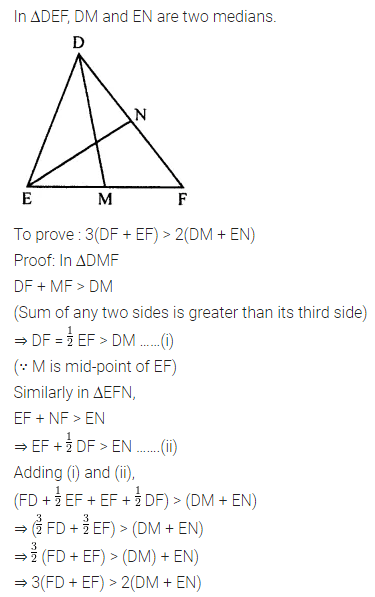Question 2.
In a ∆ABC, medians AD, BE and CF intersect each other at point G. Prove that 3(AB + BC + CA) > 2(AD + BE + CF).
Solution: